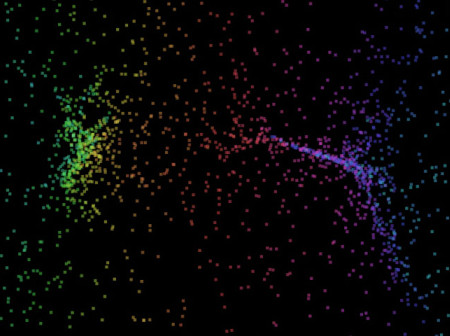# Encoding Text in UTF-8 – How Unicode Works (Part 2)

In part 1 of this article I covered the idea of creating character sets, and different strategies for encoding them. The article covered UTF-32 and UTF-16 encodings with the benefits and drawbacks of each. However, for most documents, UTF-8 encoding is the most popular by far, but is more complicated in its implementation.

For a quick re-cap, a code point is a base unit of meaning in the Unicode. A code point can represent a single letter, like “a”, but it’s not always this simple. In more complex cases code points can combine together to represent graphemes. Code points can also define other behaviors beyond just simple letters displayed to the screen.

However, what we’re interested in with UTF-8 is to be able to store a code point in a variable list of single byte (`u8`) values. A byte is made up of 8 bits.

``````                    Bits       Bytes     Max Code Points   Visual Bit Size
--------   -------   ---------------   --------------------------------------
Bytes for Unicode:  21 bits                   2,097,152                0_0000_0000_0000_0000_0000
UTF-32 (u32):  32 bits    4 bytes    4,294,967,296   0000_0000_0000_0000_0000_0000_0000_0000
UTF-16 (u16):  16 bits    2 bytes           65,536                       0000_0000_0000_0000
UTF-8   (u8):   8 bits    1 byte               256                                 0000_0000
``````

UTF-32 easily fits the potential 2,097,152 code points into a single `u32`. In part 1 I showed how UTF-16 can store the full code point range using surrogate pairs (two adjacent code points) to represent a higher plane code point. Code points in the basic multilingual plane (which tend to be more common) can be represented by a single `u16`, and then higher plane code points require two `u16` values.

## Single byte values and ASCII

UTF-8 is a variable length encoding. This means that each code point takes one or more bytes (`u8` values) to be encoded. The easiest code points to encode in UTF-8 are the ASCII range values, or officially in unicode the “C0 Controls and Basic Latin” code block. This range of values takes 7 bits and can represent the first 128 code points.

Consider this sampling of code points from this block.

``````Codepoint  Binary      Letter   Name
=========  =========   ======   =============================
U+0000     0000_0000   NUL      Null character (control code)
U+0021     0010_0001   !        Exclamation mark
U+0022     0010_0010   "        Quotation mark
U+0027     0010_0111   '        Apostrophe
U+0028     0010_1000   (        Left parenthesis
U+002B     0010_1011   +        Plus sign
U+002C     0010_1100   ,        Comma
U+0042     0100_0010   B        Latin Capital letter B
U+0048     0100_1000   H        Latin Capital letter H
U+006B     0110_1011   k        Latin Small Letter K
U+0079     0111_1001   y        Latin Small Letter Y
U+007B     0111_1011   {        Left Curly Bracket
U+007E     0111_1110   ~        Tilde
U+007F     0111_1111   DEL      Delete (control code)
``````

The common feature here is that the most significant bit is always 0. This serves as the binary signal that the byte represents a single code point, and does not require any more bytes. This is a neat property in that the UTF-8 encoding of ASCII characters is fully backwards compatible with ASCII encoded characters.

## Time to get variable

The next step here is to consider what happens if the most significant bit is 1. This signals to the encoding that the code point uses a variable length encoding. For variable length encodings, the first byte will include information about how many bytes it takes to represent the code point. Let’s ignore the trailing bytes for now, and only look at the first byte.

`110x_xxxx` Signals that the code point requires 2 bytes to represent it.
`1110_xxxx` Signals that the code point requires 3 bytes to represent it.
`1111_0xxx` Signals that the code point requires 4 bytes to represent it.

The variable length of bytes after this leading byte all start with a `10xx_xxxx`. Given this pattern, let’s buid out a table and analyze how many bits that leaves us to represent a codepoint. The x characters represent the available bits for encoding data.

``````Byte Count   Bytes and Bit Pattern                    Available bits
==========   ======================================   ==========================   =======
1            0xxxxxxx  -         -         -                            xxx_xxxx    7 bits
2            110xxxxx  10xxxxxx  -         -                       xxx_xxxx_xxxx   11 bits
3            1110xxxx  10xxxxxx  10xxxxxx  -                 xxxx_xxxx_xxxx_xxxx   16 bits
4            11110xxx  10xxxxxx  10xxxxxx  10xxxxxx   x_xxxx_xxxx_xxxx_xxxx_xxxx   21 bits
``````

21 bits can represent a maximum of `2,097,152` code points given that `2^21` is `2,097,152`. In hex this number is 0x20_0000. The largest code point in Unicode is U+10_ffff, which means we’ve found the number of bits needed to represent all of the code points.

Doing the math in the table below shows the code point ranges, and the minimum bytes needed for a given range of Unicode code points.

``````Byte Count   Code Point Range    Math
==========   ==================  =========================
1            U+0000  - U+007F     7 bits  2^7  =      0x80
2            U+0080  - U+07FF    11 bits  2^11 =     0x800
3            U+0800  - U+FFFF    16 bits  2^16 =  0x1_0000
4            U+10000 - U+10FFFF  21 bits  2^22 = 0x20_0000
``````

## Exploring the bytes on your own.

The following input can be used to test what the encoding of different text looks like with UTF-8. Feel free to change the contents and explore it.

## Variable length leaves room for bad encodings

At this point the leading bits give a good indication of the expected number of bytes. However these encodings could be incorrect, giving bad (or potentially dangerous) results. The table below shows various incorrect combinations of bytes.

❌ An ASCII byte followed by a variable length byte:
`0xxxxxxx 10xxxxxx`

❌ The first byte indicates that there is one more byte, however there are actually 2.
`110xxxxx 10xxxxxx 10xxxxxx`

❌ The first byte indicates that there are two more byte, however the next byte is in the ASCII range.
`1110xxxx 0xxxxxxx`

## Surrogate pairs are not valid in UTF-8

The other point of failure for UTF-8 encoding is that UTF-16 surrogate pairs are not valid in UTF-8. As a reminder, these surrogate pairs are a way to encode higher plane values into UTF-16. However, in UTF-8, there is enough encoding space with the variable length of bytes to encode the full Unicode code point space. Because of this, these surrogates are considered invalid.

## Iterating over characters

One thing striking about the Rust language, is that the basic string types are all valid UTF-8. This is in contrast with the JavaScript language, where all of the strings are UTF-16. Iterating over UTF-16 strings mostly works without worrying too much about it.

``````// The string is UTF-16 encoded:
var string = "¡Hola! ⇨ 👋";

// Iterate over code units/
for (let i = 0; i < string.length; i++) {
const character = string[i];
console.log("Character: ", character);
}

// Character:  ¡
// Character:  H
// Character:  o
// Character:  l
// Character:  a
// Character:  !
// Character:
// Character:  ⇨
// Character:  �    <- U+D83D  The 👋 is made up of a surrogate pair
// Character:  �    <- U+DC4B
``````

As can be seen with the emoji, the iteration breaks when dealing with higher plane values that require surrogate pairs to represent them. In UTF-8, the encoding is much more variable, and iterating over the bytes directly would only work on ASCII-encoded text.

## The Rust code point iterator

The following code examples are written using the Rust programming language. I attempted to write it in such a way that you don’t have to be familiar with Rust, and hopefully it will read like pseudo-code.

💡 Tip: Paste the code examples into the main() function in play.rust-lang.org/ and run them directly in your browser.

Now, iterating over the bytes of a UTF-8 string is probably not all that useful:

``````// Create a new string, using the example from above and print it out.
let string = String::from("¡Hola! ⇨ 👋");
println!("Bytes for {:?}", string);

// Use a byte iterator in a for loop.
for byte in string.as_bytes() {
// Print out each byte in hex on the same line.
print!("{:x} ", byte);
// c2 a1 48 6f 6c 61 21 20 e2 87 a8 20 f0 9f 91 8b
}
``````

Run example

But iterating using the built-in char iterator is a lot more useful.

``````// Create a new string, using the example from above and print it out.
let string = String::from("¡Hola! ⇨ 👋");
println!("Chars for {:?}", string);

// Use a code point (chars) iterator in a for loop.
for ch in string.chars() {
// Print out each char on the same line.
print!("{:?} ", ch);
// '¡' 'H' 'o' 'l' 'a' '!' ' ' '⇨' ' ' '👋'
}
``````

Run example

Since the bytes are variable length compared to the number of code points in the string, Rust provides a way to get either the underlying bytes, or an iterator for the code points (technically scalar values, more on this later). One key problem with working with UTF-8 is that you can’t just index directly into the data and know that you will be on a code point boundary, or how many code points in you are. Iteration is the key for working with code points.

So how does Rust build a code point iterator? Let’s build our own!

## Building a code point iterator.

First off? What exactly is a `char` in Rust? Rust provides a `std::mem::size_of` function which will give you the size of a type in bytes.

``````println!("Size of char: {:?} bytes", std::mem::size_of::<char>());
// Size of char: 4 bytes
``````

The char is 4 bytes, which is more than adequate to hold any Unicode code point. This type can then hold our decoded UTF-8 code point. Rather than use the char, we’ll use a raw u32 that matches the size of a char. Now it would be more Rust-like to have a true iterator here, but for the simplicity of this code example we will push the `u32`s on to a vector. To keep this example

``````let string = "¡Hola! ⇨ 👋";

// Create a mutable Vector, this allows us to push chars onto it.
let mut ascii_code_points: Vec<u32> = Vec::new();

// Use a byte iterator in a for loop.
for byte in string.as_bytes() {
// Check to see if the leading bit is 0.
if byte >> 7 == 0 {
// This is a single byte ASCII character.
ascii_code_points.push(
// `as u32` here will pad the byte with zeros to fit into the 4 byte char.
*byte as u32
);
}
}

// Prints: "The ASCII code points are [48, 6f, 6c, 61, 21, 20, 20]"
println!("The ASCII code points are {:x?}", ascii_code_points);
``````

Run example

This example is pretty basic and limited so far, as it does not handle the variable length bytes. The following code is a bit larger piece of code. I’ve liberally added code comments to the code. The Rust is written in a way to hopefully be more readable to a casual programmer who does not have Rust experience. I favored simple code that is full of comments rather than focusing on writing idiomatic Rust.

``````/// Take a string, and return the code points as `u32`s. The bytes of the string
/// are encoded as UTF-8, and so we must re-assemble the code points by processing
/// the encoding.
///
/// Normally in Rust, you would use the built-in `string.chars()` method, but let's
/// build our own implementation.
///
/// This function takes a "string slice", which is a view into the string, returns
/// a vector of u32 values.
fn get_code_points(string: &str) -> Vec<u32> {
// These are the code points we will return.
let mut code_points: Vec<u32> = Vec::new();

// This next line turns the string slice into an iterator of bytes.
let mut byte_iterator = string.as_bytes().iter();

// Use an infinite loop so we can manually iterate over the bytes.
loop {
// Move the byte iterator forward.
let maybe_first_byte = byte_iterator.next();
if maybe_first_byte.is_none() {
// There are no more bytes, break the loop.
break;
}

// There is a byte, unwrap it so we can use it below.
let first_byte: u8 = *maybe_first_byte.unwrap();

// We need to figure out the bytes for the final u32 code point value. These
// bytes are stored in "little endian" order, where the least significant bytes
// are first. For example, an ASCII letter would only be stored in the first
// byte of this array.
//
// Note that the 4th byte will never be written to, and will always be 0, since
// the code points fit in only 3 bytes.
let mut code_point_bytes = [0, 0, 0, 0];

// This is a helper function to get the next "trailing byte" from the UTF-8 string.
// By trailing byte, I mean the bytes that start with 0b10xx_xxxx. Feel free to
// come back to this function after reading the `if` statements below.
let mut get_trailing_byte = || {
// There must be another byte, ore else the ".expect()" panics with the error
// message.
let byte = byte_iterator
.next()
.expect("Unable to find a trailing byte");

// Make sure the byte has the proper start with 0b10xx_xxxx by shifting all
// the bytes over to the right. If this fails, then our Rust program will
// panic and exit.
assert!(byte >> 6 == 0b10, "Expected a trailing byte");

// Only return the data part by remove the 0b10 at the beginning.
return byte & 0b0011_1111;
};

// Now match which type of byte this is. The strategy here is the `if` check
// will shift the bits over in the byte to the right using the >> operator. It
// will then check to see if the bit prefix matches one of the 4 patterns:
//
//  0b0xxx_xxxx  1 byte
//  0b110x_xxxx  2 bytes
//  0b1110_xxxx  3 bytes
//  0b1111_0xxx  4 bytes
//
// Next the code will use the & operator to mask the byte to extract the the
// encoded data. This encoded data will be split up and placed in the correct
// bytes in the `code_point_bytes`.

if first_byte >> 7 == 0b0 {
// This is an ASCII character since it starts with 0b0xxx_xxxx. It can be
// stored literally. There is only one byte here. This is the simplest case.
code_point_bytes = first_byte;
} else if first_byte >> 5 == 0b110 {
// There are two bytes here, since it starts with 0b110x_xxxx. Store only
// the encoded data in a and b.
let data_a = 0b00011111 & first_byte;
let data_b = get_trailing_byte();

// This code shifts around the encoded bits into the proper byte order. It's
// doing bit manipulation which can be somewhat hard to describe. Essentially
// its extracting the relevant encoded data, and putting it into the final
// form for the u32.
code_point_bytes = data_b | (data_a << 6);
code_point_bytes = data_a >> 2;
} else if first_byte >> 4 == 0b1110 {
// There are three bytes here, since it starts with 0b1110_xxxx.
let data_a = 0b00001111 & first_byte;
let data_b = get_trailing_byte();
let data_c = get_trailing_byte();

// This code shifts around the encoded bits into the proper bytes. Note that
// even though the UTF-8 requires 3 bytes, the final encoding only needs
// 2 bytes.
code_point_bytes = data_c | (data_b << 6);
code_point_bytes = (data_b >> 2) | data_a << 4;
} else if first_byte >> 3 == 0b1111_0 {
// There are four bytes here, since it starts with 0b1111_0xxx.

// Notice how there are only 3 bits of encoded information in this first byte.
let data_a = 0b00000111 & first_byte;
let data_b = get_trailing_byte();
let data_c = get_trailing_byte();
let data_d = get_trailing_byte();

// This code shifts around the encoded bits into the proper bytes. Note that
// even though the UTF-8 requires 4 bytes, the final encoding only needs
// 3 bytes.
code_point_bytes = data_d | (data_c << 6);
code_point_bytes = (data_c >> 2) | data_b << 4;
code_point_bytes = (data_a << 2) | data_b >> 4;
} else {
// This byte was badly encoded. The panic here will exit the program.
panic!("Found an unexpected byte.");
}

// Push on the assembled u32 value from the "little endian" ordered bytes.
code_points.push(u32::from_le_bytes(code_point_bytes));
}

return code_points;
}

// The main function is the entry point to the program, so we can test the function
// we wrote above.
fn main() {
// This is the same string from the examples above.
let string = "¡Hola! ⇨ 👋";

// Run the custom get_code_points function.
let code_points = get_code_points(string);

// Prints: "Code points: [a1, 48, 6f, 6c, 61, 21, 20, 21e8, 20, 1f44b]"
println!("Code points: {:x?}", code_points);

// We can also view these as individual "chars" by mapping the u32 to a char type.
let chars: Vec<char> = code_points
.iter()
.map(|val| char::from_u32(*val).expect("Failed to convert u32 to a char"))
.collect();

// Prints: "The chars: ['¡', 'H', 'o', 'l', 'a', '!', ' ', '⇨', ' ', '👋']"
println!("The chars: {:?}", chars);

// Let's compare our custom implementation with the standard library by using the
// `string.chars()` method. The `char` type will need to be mapped to u32.
let std_code_points: Vec<u32> = string.chars().map(|char| char as u32).collect();

// Prints: "The chars are [a1, 48, 6f, 6c, 61, 21, 20, 21e8, 20, 1f44b]"
println!("Code points: {:x?}", std_code_points);

// Assert that our implementation matches.
assert_eq!(
code_points, std_code_points,
"The custom code point implementation matches the std implementation."
);
}
``````

Run example

That was a bit of code, congrats on getting through it. This shows that iterating over UTF-8 code involves a bit more complexity compared to other encodings. There is a trade-off between the simplicity of the encoding and implementation and the memory size of the encoded text.

One thing that we did not handle in our code was checking for high and low surrogates. Because of this, we made a “Unicode code point” iterator, but not a “Unicode scalar value” iterator. The Rust docs for the primitive type `char` has the following line:

The char type represents a single character. More specifically, since ‘character’ isn’t a well-defined concept in Unicode, char is a ‘Unicode scalar value’, which is similar to, but not the same as, a ‘Unicode code point’.

This should makes sense because a Unicode scalar values is defined as.

Any Unicode code point except high-surrogate and low-surrogate code points.

The surrogate code points are only useful in the UTF-16 encoding scheme.

## What’s next after code point iteration?

Code points are definitely more useful to iterate over compared to raw UTF-8 encoded bytes. However, code points aren’t always the boundary that we need for expressing what is drawn to a screen. It can take combinations of code points to express a character such as diacritical marks or emojis. Check out my article on diacritical marks for a full deep dive into this topic.

In order to iterate over other boundaries, we require more complicated text segmentation algorithms. These algorithms require more information like Unicode property tables to understand the meaning of code points. This requires data tables that must be kept up to date, and is beyond the scope of this article. There are ICU libraries for handling these more complicated iterators, and bindings and implementations available in different languages. For an example to play around with, there is the `unicode_segmentation` crate which provides iterators for grapheme clusters (essentially characters you see on the screen), words, and sentences.

## UTF-8

UTF-8 is the most common encoding on the web by far. From Wikipedia:

UTF-8 has been the most common encoding for the World Wide Web since 2008. As of November 2021, UTF-8 accounts for on average 97.5% of all web pages

Hopefully this article helps shed some light on how this encoding works, and some of the challenges with working with a variable length encoding scheme.

More From writing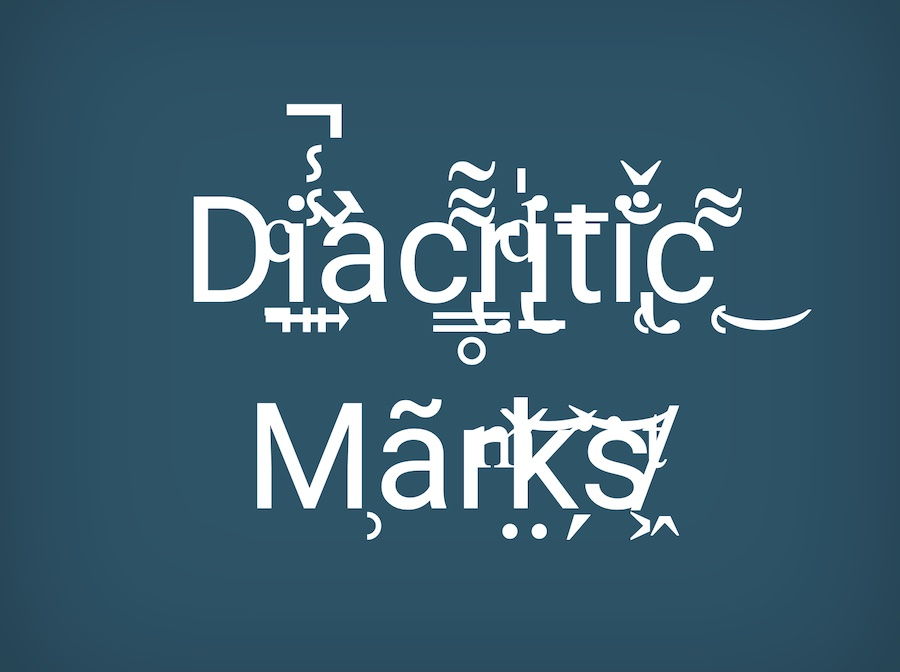## Diacritical Marks in Unicode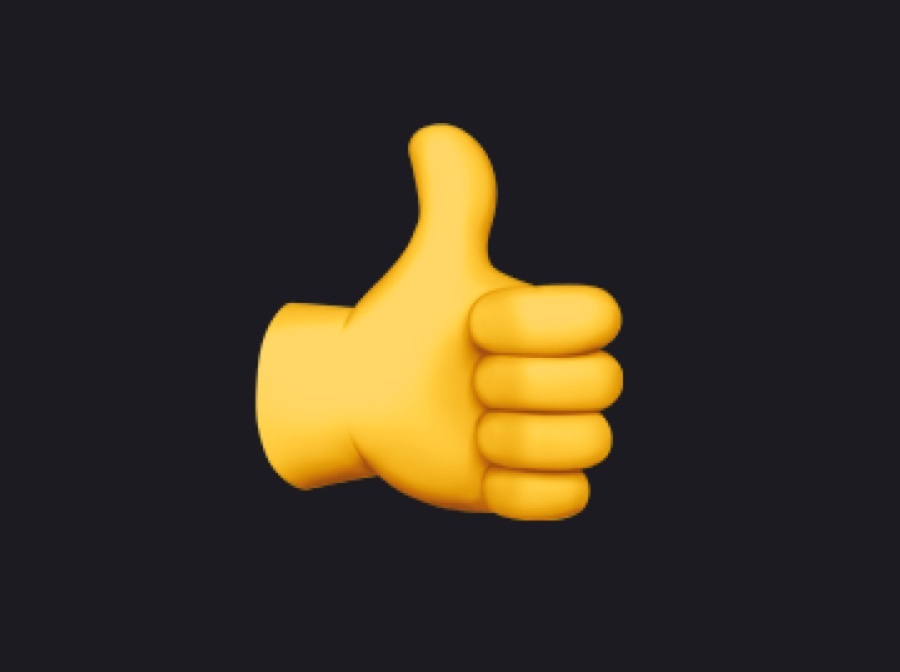## Encoding Text, UTF-32 and UTF-16 – How Unicode Works (Part 1)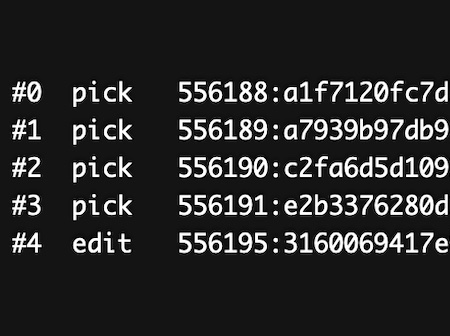## Better Code Reviews with Mercurial History Rewriting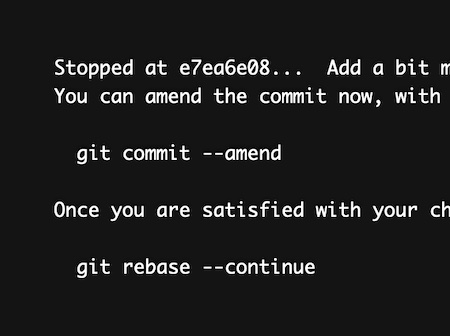## Better Code Reviews with git History Rewriting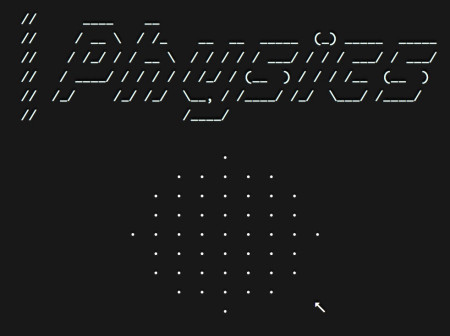## Drawing ASCII Art to Test a Physics System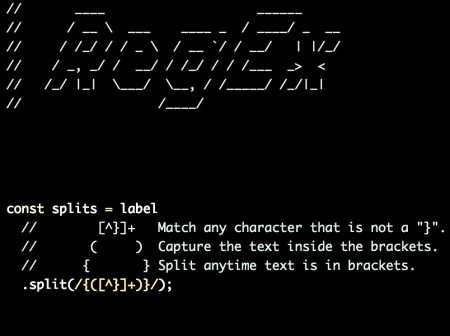## Documenting Regex with ASCII Art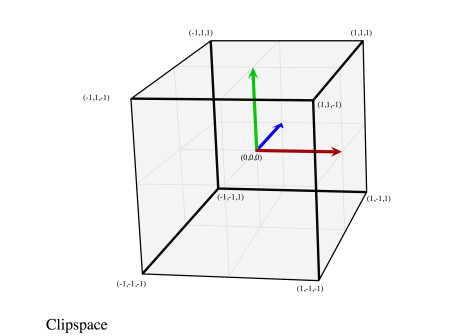## WebGL Model View Projection